(This web page was created using latex2html. It contains numerous inline gifs. To view it properly, use a graphical browser such as Netscape or Explorer; a text browser such as Lynx will not suffice.)

# AN OPEN LETTER

To: The authors of calculus textbooks

From: Several authors of more advanced books and articles --

Robert Bartle, USA <mth bartle@emuvax.emich.edu>
Ralph Henstock, Ireland <r.henstock@ulst.ac.uk>
Jaroslav Kurzweil, Czech Republic <kurzweil@mbox.cesnet.cz>
Eric Schechter, USA <schectex@math.vanderbilt.edu>
Stefan Schwabik, Czech Republic <schwabik@beba.cesnet.cz>
Rudolf Výborný, Australia <R.Vyborny@mailbox.uq.edu.au>

Subject: Replacing the Riemann integral with the gauge integral

It is only an accident of history that the Riemann integral is the one used in all calculus books today. The gauge integral (also known as the generalized Riemann integral, the Henstock integral, the Kurzweil integral, the Riemann complete integral, etc.) was discovered later, but it is a "better" integral in nearly all respects. Therefore, we would like to suggest that in the next edition of your calculus textbook, you present both the Riemann and gauge integrals, and then state theorems mainly for the gauge integral.

This switch would only require altering a few pages in your calculus book. Any freshman calculus book is devoted almost entirely to derivatives and antiderivatives -- how to compute them and how to use them; that material would not change at all. The only changes would be in the more theoretical sections of the book -- i.e., the definitions and theorems -- which take up only a few pages.

The reasons for making this change are twofold: (i) It would actually make some parts of your book more readable. Some definitions and theorems can be stated more simply (and more strongly) if the gauge integral is used instead of the Riemann integral. This is particularly true of the Second Fundamental Theorem of Calculus, discussed below. (ii) It would be a better preparation for the handful of calculus students who will go on to higher math courses. The gauge integral is far more useful than the Riemann integral, as a bridge to more advanced analysis.

The idea of introducing the gauge integral to college freshmen is not entirely new; it was promoted, for instance, in the paper "The Teaching of the Integral" by Bullen and Výborný, Journal of Mathematical Education in Science and Technology, vol. 21 (1990). However, we feel that the idea deserves wider promotion; hence this letter.

Introduction to the integral. If you are not already familiar with the gauge integral, we would recommend the article by Bartle in the American Mathematical Monthly, October 1996; it provides a quick introduction to the subject and gives most of the main references. Perhaps a second introduction would be the book of DePree and Swartz (Introduction to Real Analysis, Wiley, 1988); it is written for advanced undergraduates and is probably the most elementary among the currently available introductions to this subject. Professors Bartle and Schechter have volunteered to make themselves available, at least to some extent, to answer further questions you may have about this subject.

The definition. The gauge integral is a very slight generalization of the Riemann integral. Instead of a constant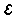and a constant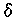, it uses a constantand a function. The two definitions can be formulated so that they are nearly identical, and then placed side by side. This would be helpful to the teachers who are using the book and learning about the gauge integral for the first time.

We think that the slight increase in the complexity of the definition will make little difference to the students learning from the book. It has been our experience that, for the most part, freshman calculus students do not fully grasp the definition of the Riemann integral anyway; it is just too complicated for students at that level. The definition of the Riemann integral is included in a calculus book more for completeness and integrity than for teaching. The few students who have enough mathematical maturity to grasp the definition of the Riemann integral will probably have no greater difficulty with the gauge integral, and they will benefit from being exposed to this concept in their calculus book.

Existence and nonexistence of integrals. In recent calculus books it is customary to state without proof that continuous functions and monotone functions on a compact interval are Riemann integrable. The omission of the proofs is unavoidable -- a proof would involve the completeness of the reals, uniform continuity on compact intervals, and other notions that are far beyond the reach of freshmen.

Any Riemann integrable function is also gauge integrable. Many more functions are gauge integrable, as will be evident later in this letter; see especially our remarks about the Dominated Convergence Theorem.

It is customary to give the characteristic function of the rationals as an example of a function that is not Riemann integrable. Most students don't fully understand this example, because the characteristic function of the rationals is too unlike familiar examples to appeal to the intuition of most students. Still, it is useful to include such an example in the book, because the student comes away convinced (perhaps through trust rather than through understanding) that not every bounded function on a closed bounded interval is Riemann integrable.

A book covering the gauge integral might still include the characteristic function of the rationals, as an example of a bounded function that is gauge integrable but not Riemann integrable on a closed bounded interval.

Ideally, one would like to also include a concrete example of a bounded function that is not gauge integrable on a closed bounded interval. Even if it is complicated, the presence of such an example would convince the student (again, through trust) that not every function is gauge integrable. Unfortunately, there are no concrete examples, as explained later in this letter. That is both a strength of the theory (essentially all functions are gauge integrable) and a weakness of the theory (it is hard to convey the concept of the few functions that are not gauge integrable).

The book might include a statement something like this: "Heuristically, every bounded function that one is likely to encounter in applications is gauge integrable on any closed bounded interval. Although it is possible to prove mathematically that there exist bounded functions that are NOT gauge integrable on closed bounded intervals, such functions do not arise in practical applications. We shall discuss this further, briefly, in the appendix." Some possibilities for the appendix are discussed later in this letter.

The first fundamental theorem of calculus: derivatives of integrals. There are two versions of this theorem -- an elementary version and a more advanced version. The elementary version is this:

Supposeexists. Then F is continuous. Moreover, at every t where f(t) is continuous, the derivative F '(t) exists and equals f(t).
We don't have to assume f is continuous everywhere. The theorem is not hard to prove. A remark might be made that "a more advanced and stronger version of this theorem can be found in the appendix." The more advanced version says:
Supposeexists. Then F is continuous. Moreover, at almost every t, the derivative F '(t) exists and equals f(t).
("Almost every" would have to be defined in the appendix.) We don't have to make any assumption at all about continuity of the integrand, f. However, this more advanced version is harder to prove; you'd have to just state it without proof.

The second fundamental theorem of calculus: integrals of derivatives. Here the gauge integral offers a big advantage over the Riemann integral. Again there are two versions. The elementary version is:

If f(t)=F '(t) exists for all t in [a,b], then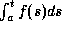exists and equals F(t)-F(a).
We don't have to make any assumption of continuity of f. The proof of this theorem is easy enough to include in a freshman calculus book. In an appendix, after introducing countable sets, you could state and prove this advanced version:
Suppose F is continuous. Also suppose f(t)=F '(t) exists for all but countably many points t in [a,b]; define f arbitrarily at those points. Thenexists and equals F(t)-F(a).
The proof is not very hard. (By the way, in this theorem, "for all but countably many points" cannot be replaced by "except on a set of measure 0"; that is shown by the Cantor Function.)

Convergence theorems. Calculus books traditionally have included the theorem that uniform limits preserve integrability and integrals. However, for the gauge integral we can state this much nicer and stronger Dominated Convergence Theorem:

Suppose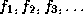are gauge integrable functions on [a,b], converging pointwise to a limit function f. Suppose that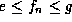for all n, where e and g are gauge integrable functions. Then f is gauge integrable, and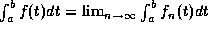.
Thus, under rather simple and mild assumptions, pointwise limits preserve integrability and integrals. This theorem can be used to demonstrate the integrability of a very wide class of functions.

The Monotone Convergence Theorem and Dominated Convergence Theorem could be stated without proof, and then examples and exercises can be given. Applications can be found in any book on the Lebesgue integral.

(Actually, the Monotone and Dominated Convergence Theorems are also true, in appropriate forms, for Riemann integrals. Some references can be found in a paper by Simons, Math. Intelligencer 17 (1995), 67-70. However, the proofs in that setting are not much shorter than the proofs in the more general setting of gauge or Lebesgue integrals, and so there is not much reason to bother with them in an introductory course.)

Improper integrals. A separate definition is not required. A statement such as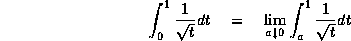becomes a theorem, rather than a definition of the left side of the equation. Admittedly, we still end up using the right side of the equation as a method for computing numerical values, but it is conceptually advantageous to have this equation as a theorem instead of a definition.

A separate definition can also be avoided for integrals over unbounded intervals. A statement such asbecomes a theorem, rather than a definition of the left side of the equation, if the definition of the integral is formulated as in McLeod's book -- i.e., unbounded subintervals make 0 contribution to the approximating Riemann sum. (Admittedly, this may be counterintuitive to beginners, so it is perhaps debatable whether this more general definition is desirable in a calculus textbook.)

Integration formulas. Any calculus book must include the substitution rule (i.e., change of variables or reparametrization in integrals) and integration by parts. Usually these are stated as computational formulas, with the regularity hypotheses glossed over. If one does wish to state the regularity hypotheses explicitly, those hypotheses can be weakened slightly by switching from Riemann integrals to gauge integrals.

Appendices. At the end of your book you may wish to add a few pages of material which has not customarily appeared in calculus books. This is more for the benefit of the teachers and occasional gifted students than for the typical student. Here is an outline of some suggested material:

Countability. It would be helpful to discuss the difference between countable and uncountable sets. Examples would include the fact that the integers and the even integers are equipollent; Cantor's proofs that the rationals are countable but the reals are not. The union of countably many countable sets is countable. The gauge integral of the characteristic function of any countable set is 0. These results are not hard to prove.

Anticipating results below about measure, you might also prove that the Cantor set is uncountable. (To simplify the exposition, remove the middle 8/10 instead of the middle 1/3; then you can use decimal expansions instead of ternary expansions.)

A theorem of Cantor states that any set has smaller cardinality than its power set. The proof is is actually very short and easy, except that it is rather abstract.

Measure. A set is measurable if and only if its characteristic function is gauge integrable; then the measure of the set is equal to the value of that integral. Easy examples show that this extends the notion of length, and gives us a notion of "size" for a very large class of subsets of the real line. Admittedly, defining size in terms of integrals is conceptually backward -- many mathematicians find it more intuitive to define integrals in terms of measures -- but for purposes of your calculus book, this approach saves several pages.

Some basic properties can be stated, with or without proof. The union of countably many disjoint measurable sets is measurable, and its measure is the sum of their measures; etc. If a function vanishes outside a set of measure 0, then that function is gauge integrable and its integral is 0. Any countable set has measure 0. The Cantor set is an uncountable set with measure 0, since it is contained in simpler sets with arbitrarily small positive measure.

Unmeasurable sets. Vitali's classical proof of the existence of an unmeasurable set is fairly short and elementary. It can be found in any book on Lebesgue integration. A sketch of the proof might be useful in the appendix to a calculus book. The typical calculus student would not understand the proof, but a few students might understand a few parts of the proof. In any case, the student would go away believing (perhaps on trust rather than on understanding) that there do exist unmeasurable sets.

Vitali's proof uses the Axiom of Choice in a fairly simple and intuitive way. The expositor can point out the Axiom of Choice when it is used, and emphasize that the proof is not constructive. It can be stated that there are no constructive ways of obtaining unmeasurable sets. (The proof of the fact that there are no constructive ways is rather difficult; it involves much set theory and logic. It is discussed in part of Schechter's book, Handbook of Analysis and its Foundations, Academic Press, 1996.) Thus, we know that there exist bounded functions that are not gauge integrable on a closed bounded interval, but we cannot produce explicit examples of such functions.

In summary, we feel that the changes in the calculus book would not be major but would improve the teaching of calculus. We invite your questions on these matters.

Sincerely,

Robert Bartle, Ralph Henstock, Jaroslav Kurzweil, Eric Schechter, Stefan Schwabik, and Rudolf Výborný

(This letter is being distributed to publishers' representatives at the Joint Mathematics Meetings in San Diego, California, in January 1997. We may also try to publish it and/or distribute it in some other fashion in the near future, perhaps with slight modifications and/or more signatures.)